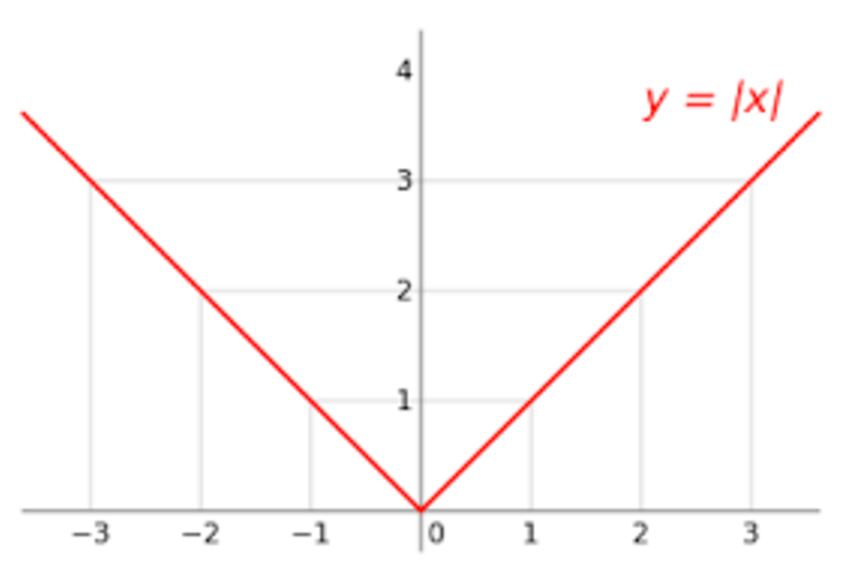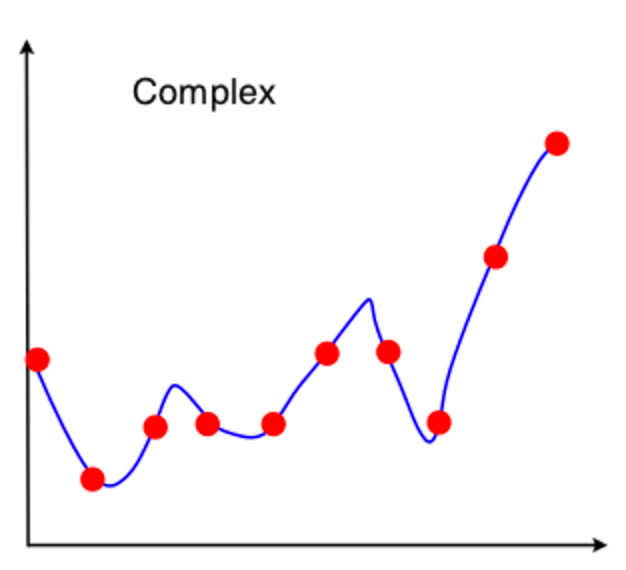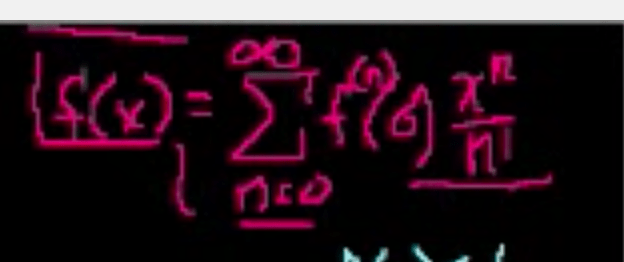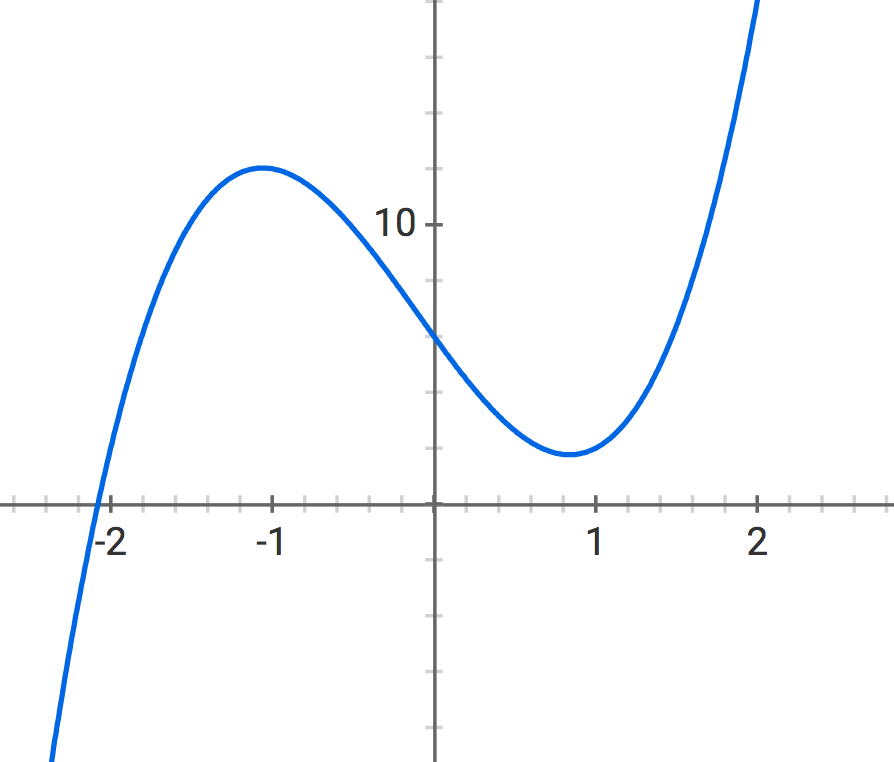# Machine Learning Intuition: Understanding Taylor Series Approximation

We have talked before about the intuition behind cost function optimization in machine learning. We took a look at where cost functions come from and what they look like. We also talked about how one might get to the bottom of one with gradient descent.

When you open a machine learning textbook, you’ll see much more math than we used in that introduction. But the math is, in fact, important; the math gives us tools that we can use to quickly find the minima of our cost functions.

We talked about derivatives in the last post, and we’re talking about Taylor series in this post. Both of these tools come from calculus and help us identify where to find the minima on our cost functions.Why do we even need the Taylor Series?

We used derivatives in the last post (called differentiating the function) to find flat points on cost functions that might minimize the cost. But not all functions are differentiable everywhere. Take, for example, the absolute value function:This thing is the bane of mathematicians’ existence. Look at it! There’s it’s global minimum, clear as day, at x = 0, but we can’t differentiate the function at x = 0 because it’s pointy there rather than flat. In this case, we can take a darn good guess because we can see the minimum with our eyeballs. But what if we’re talking about a more complex function?Not this kind of complex. I mean dimensionally complex. Suppose you’re trying to fit a function with more than 3 input variables…as you often will when fitting a regression to a dataset. You only have 3 dimensions in space, so now you can’t represent all your variables in a picture. There’s a whole field of machine learning devoted to dimensionality reduction specifically to help fit datasets into the strict parameters of what our puny brains can visualize.

But because of those limits, we need the ability to minimize functions that we can’t differentiate everywhere and can’t visualize. So we pick a spot where we can differentiate the function, and we use it to find an approximation of where the function will be at the point that we care about…like a minimum.

Enter Taylor Series!

These videos from Khan Academy really helped me to understand how Taylor Series work work: one, two, three. That having been said, the videos are fairly heavy on notation. That can be a hangup because most people don’t read things that look like this every day:Let’s see if we can understand the Taylor series approximation with a little less notation and a small number of dimensions, step by step.

Suppose we have this function to approximate: f(x) =3x3 + x2 – 8x + 6. It looks like this:This function is not so complicated, but we’ll use it to demonstrate how the Taylor series approximates what’s going on.

Suppose we want to know approximately what is happening with this function around x=1:For this function we could differentiate at 1, and we could also plug 1 into f(x) =3x3 + x2 – 8x + 6. But we’re using this function to show how the Taylor Series works, so when we do have to break down a function we understand why. This same technique could be used to find out an approximate value for f(x) = 2|x| + 6 at x = 0, where it is not differentiable, by using the position and local derivative of that function at x = -1, where the function is differentiable. It could also be used for more complex, not-entirely-differentiable functions that we’d have trouble imagining in our heads.

The Taylor Series is a sum of a series of derivatives from the original function. The way it works is that we can calculate approximately where a function lies at one point based on where it lies at another point, taking into account its derivatives to figure out how much it changes from our anchor point to the point we want to find. In our case we will use an anchor point of zero, because it’s easy to multiply things by zero so lots of terms cancel out.

So we’ll use this variant of the Taylor series, which plugs in our anchor point of zero for everything:When you do that, it’s called a Maclaurin Series.

We’ll approximate where the function is at 1 by finding the first term in the Taylor series: where is the function at zero?

f(1) ≈ 3(0)3 +(0)2 – 8(0)+ 6 = 6

We can look at the graph and see that 6 is not a great approximation of where this function is at 1. So let’s calculate the next term of the series, too: the derivative of the function multiplied by the difference between our target point and our anchor point, divided by one factorial (which is just 1).

f(1) ≈ (3(0)3 +(0)2 – 8(0)+ 6) + ((9(0)2  + 2(0) – 8)*((1-0)/1)) = 6 + -8*1 = 6 – 8 = -2

This approximation is also not excellent, but it’s actually closer to the real answer than 6. Our estimate of where the function is at 1 dropped a lot because, at 0 where we are anchored, our function is going down pretty steeply. So the next term, which is based on that slope, brings down our estimate a lot based on where the function looks like it’s going at zero.

Let’s do another term to approximate even better. The next term takes the derivative of the derivative of the function, multiplied by the difference between our target point and our anchor point squared, divided by two factorial.

f(1) ≈ 6 – 8+ ((18(0)  + 2)*((1 – 0)2/2)) = 6 – 8 + (2*1/2) = 6 – 8 + 1= -1

This approximation is even closer. The slope is very negative at 0, but it’s getting more positive (it’s concave up). So this next term adjusts for that by bringing our estimate higher again.

Let’s do another term to approximate even better.

f(1) ≈ 6 – 8 + 1 + ((18)*((1 – 0)3/3*2*1)) = 6 – 8 + 1 + (18*1/6) = 6 – 8 + 1  + 3= 2

Hey, this approximation is getting pretty good now!So, speeding things up a bit, suppose we were looking for the minimum of f(x) = 2|x-2| + 6. We know this is not differentiable everywhere, but it is differentiable at x = 0. At that point the function is going down and the derivative is -2. Let’s apply a Taylor series at that point to figure out the value at our suspected minimum, x = 2.

f(2) ≈ (2|0-2| + 6)  = 2*2 + 6 = 10

f(2) ≈ (2|0-2| + 6)  + (-2) (2-0/1) = 10 + -4 = 6

Which is, in fact, the minimum!

It’s possible to look up even more complex examples where we can use the Taylor Series to sneak up on solutions that we cannot find directly. I’ll leave that as an exercise for you.

If you’re struggling to understand the notation, do not fret. It takes time to get used to, and it helps to go over it repeatedly with time, sleep, and other activities in between your review periods. Once that understanding of the notation starts to gel, you’ll find that the mud clears significantly and you can see what we’re doing with the Taylor Series much more clearly.

1.Michael Reinhard says:

Yes! Where have you been all my life. It is like you were listening in my head when I was reading Jeremy Watt’s book as all the questions occurred to me, like, “Why do we want to use a Taylor Series in the first place?” This is very helpful. Thank you!

2.Hwang Daniel says:

Thanks!

3.machinelearning08 says:

Explained very well. Thanks

This site uses Akismet to reduce spam. Learn how your comment data is processed.